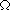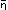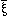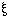HOME TOP UP PREV NEXT 0 1 2 3 GERMAN MAP      Tractatus Logico-Philosophicus 6.0

6.0

6.00
6.01
The general form of the operation' () is therefore:
[, N ()]'() (= [,, N()]).

This is the most general form of transition from one proposition to another.

6.02
And thus we come to numbers: I define
x =0'x Def. and'v'x =v+1'x Def.

According, then, to these symbolic rules we write the series x,'x,''x,'''x . . . . . as:0'x,0+1'x,0+1+1'x,0+1+1+1'x . . . . .

Therefore I write in place of "[x,,']",

"[0,v'x,v+1'x]",

And I define:

0 + 1 = 1 Def.
0 + 1 + 1 = 2 Def.
0 + 1 + 1 + 1 = 3 Def.
and so on.

6.03
The general form of the cardinal number is: [0,,+1].

HOME TOP UP PREV NEXT 0 1 2 3 GERMAN MAP      Tractatus Logico-Philosophicus 6.0